# python 倒叙 数组_python数据科学（2）Python数据科学相关编程基础（上）Python相关编程基础  本篇主要介绍 Python 的基本数据类型、数据结构； 01 基本数据类型
 名称 名称 举例 str 字符串 'a','1' float 浮点数 9.96，0.07 int 整数 9，8 bool 布尔值 True，False complex 复数 2+1j，9+0j
(1)字符串 是 Python 中最常用的数据类型。 我们可以使用引号('或")来创建字符串。 Python中单引号、双引号、三引号包括起来的都是字符串。 Python 中的字符串也支持一些格式化输出，例如换行符“\n”和\t"。 输入：
# 在Python中用引号引起来的字符集称之为字符串，比如：'hello'、"my Python"、"2+3"等都是字符串# Python中字符串中使用的引号可以是单引号、双引号跟三引号print ('hello world!')c = 'It is a "dog"!'print (c)c1= "It's a dog!"print (c1)c2 = """hello world !"""print (c2)

Python字符串运算符：+、*、[]、[ : ]、in、not in、r/R、%， 分别有不同的功能，示意如下：

# -*- coding: UTF-8 -*-a = "Hello"b = "Python" #+  字符串连接 print ("a + b 输出结果：", a + b )# *  重复输出字符串print( "a * 2 输出结果：", a * 2 )#取子字符串有两种方法，使用[]索引或者切片运算法[:]，这两个方法使用面非常广# []  通过索引获取字符串中字符print("a 输出结果：", a )# [ : ] 截取字符串中的一部分 print( "a[1:4] 输出结果：", a[1:4] )# in  成员运算符 - 如果字符串中包含给定的字符返回 Trueif( "H" in a) :    print( "H 在变量 a 中" )else :    print( "H 不在变量 a 中" )# 成员运算符 - 如果字符串中不包含给定的字符返回 Trueif( "M" not in a) :    print("M 不在变量 a 中" )else :    print ("M 在变量 a 中")# 原始字符串 - 原始字符串：所有的字符串都是直接按照字面的意思来使用，没有转义特殊或不能打印的字符。#原始字符串除在字符串的第一个引号前加上字母"r"(可以大小写)以外，与普通字符串有着几乎完全相同的语法。print (r'\n')print (R'\n')# - 字符串格式化输出 %  格式字符串print( 'The first word is %s, and the second word is %s' %(a, b))

(2)其它

#查看变量 与类型转换type(None)type(s)# 类型转换str(10086)float(10086)int('10086')
02 数据结构

students = ["ming", "hua", "li", "juan", "yun", 3]a = list(["ming", "hua", "li", "juan", "yun", 3])print (students,a)
# 1.列表切片正序的时候索引从0开始，含左不含右；倒序的时候引从1开始print(students)#打印出students列表print(len(students))#利用len()方法查询列表的长度print ('=', students)# 根据索引 查询 列表中的数据，索引是从0 开始的print ('[-4]=', students[-4])#提取倒数第4个元素 print ('[0:4]=', students[0:4])# 切片 从索引0 到 3提取list的元素print( '[4:]=', students[4:])#切片  从索引4到最后的元素全部提取 print ('[::2]=', students[::2])#切片 步长为2 print ('[0:4:2]=', students[0:4:2])# 从索引0 到 3提取list的元素，切片 步长为2print ('[-5:-1:]=', students[-5:-1:])# 从倒数第5到倒数第1提取list的元素，切片 步长为1print ('[-2::-1]=', students[-2::-1])# 从倒数第5到倒数第1提取list的元素，切片 步长为1，倒叙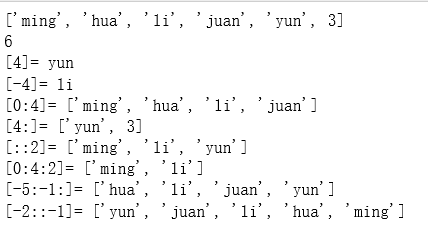#2.修改列表students = "小月"print (students)
# 3)插入元素"""append方法每次只能在末尾填入一个元素；extend方法用于在列表末尾一次性追加另一个序列中的多个值(用新列表扩展原来的列表)insert方法可在指定的位置插入一个元素；"""# In[ ]:print(students)students.append('han') # 添加到尾部print(students)students.extend(['long', 'wan'])print(students)# In[ ]:scores = [90, 80, 75, 66]students.insert(1, scores) # 添加到指定位置
# In[ ]# 4)删除元素"""pop方法在不指定参数时默认删除末尾元素，也可以指定删除某个位置的元素；remove方法删除指定的元素值；clear方法清空列表元素；del函数删除列表对象；"""# In[ ]:print (students.pop(1)) # 该函数返回被弹出的元素，不传入参数则删除最后一个元素print (students)students.remove("li")print(students)students.clear()print(students)

studentsTuple = ("ming", "jun", "qiang", "wu")studentsTupletry:    studentsTuple = 'fu'except TypeError:    print ('TypeError')'ming' in studentsTuplestudentsTuple[0:4]studentsTuple.count('ming')studentsTuple.index('jun')len(studentsTuple)

students = ["ming", "hua", "li", "juan", "yun", "hua"]studentsSet = set(students)print (studentsSet)studentsSet.add('xu')print (studentsSet)studentsSet.remove('xu')print (studentsSet)a = set("aabc")print ('a=', a)b = set("cdfm")print ('b=', b)#交集x = a & b print( 'x=', x)#并集y = a | bprint ('y=', y)#差集z = a - bprint( 'z=', z)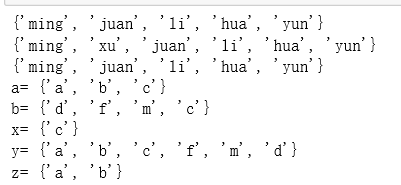k = {"name":"weiwei", "home":"guilin"}print (k["home"]) # 按照key 的值去找键的内容 # In[ ]:print( k.keys())print( k.values())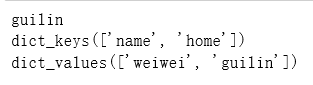a={"success":True,"reason_code":"200","reason_desc":"获取成功",   "rules":[{"rule_id":"1062274","score":7,"conditions":[{"address_a_value":       "南通市","address_a":"mobile_address","address_b":"true_ip_address","address_b_value":"南京市","type":"match_address"}]}]}# 添加、修改字典里面的项目k = {"name":"weiwei", "home":"guilin"}k["like"] = "music"  # 直接通过 集合名词[key]=value 添加k['name'] = 'guangzhou'print (k)# 判断key是否存在#要避免key不存在的错误，有两种办法，一是通过in判断key是否存在：print ('name' in k)#通过dict提供的get方法，如果key不存在，可以返回None，或者自己指定的value：print (k.get('name'))# 删除key-value元素k.pop('like')print (k)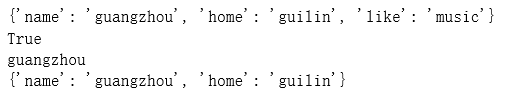## 列表、元组、集合、字典的互相转换

students = ["ming", "hua", "li", "juan", "yun", "hua"]tuple(students)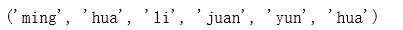#直接使用list，是将字典中的key转换为列表k = {"name":"weiwei", "home":"guilin"}list(k)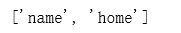#字典中的key转换为列表key_value = list(k.keys())print('字典中的key转换为列表：', key_value) #字典中的value转换为列表value_list = list(k.values())print('字典中的value转换为列表：', value_list)# zip可以将列表、元组、集合、字典‘缝合’起来zl = zip(('A', 'B', 'C'), [1, 2, 3, 4]) print (zl)print (dict(zl))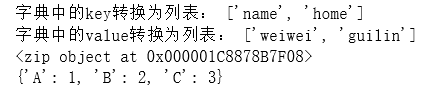jupyter代码放在GitHub上啦~ 获取地址：

https://github.com/hzrlib/python_basic

03-161万+05-091万+
03-198790
01-065万+
10-012万+
08-01133
07-142709
09-038478
01-251236
07-08409
03-058662
04-22310
08-04481
11-202368
07-221289
09-19
06-052万+
©️2020 CSDN 皮肤主题: 1024 设计师:白松林